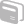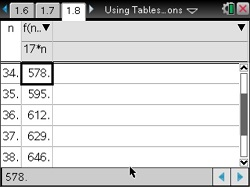# Activities

•##### Download

•• ##### Subject Area

• Math: Algebra I: Equations

• ##### Author9-12

45 Minutes

• ##### Device
•TI-Nspire™ CX/CX II
•TI-Nspire™ CX CAS/CX II CAS
• TI-Nspire™
• TI-Nspire™ CAS
• ##### Software

TI-Nspire™
TI-Nspire™ CAS

3.0

• ##### Report an Issue

Using Tables to Solve Linear Equations#### Activity Overview

Solve one-step and two-step linear equations where a and b are real numbers.

#### Objectives

• Solve "one-step" linear equations of the form x + a = b and ax = b where a and b are real numbers
• Solve "two-step" linear equations of the form ax + b = c where a and b are real numbers

#### About the Lesson

Students are given quotes verbally and asked to write each quote as a formula in the variable n. They enter the formulas into a calculator application and use a spreadsheet application to display a table of values. Students use these tables to determine the value of a quote corresponding to a given number of guests and, conversely, the number of guests that correspond to a particular quote. In this process, students solve linear equations of the form ax + b = c informally.

©Brendan Kelly Publishing, Inc. Reprinted with permission. www.brendankellypublishing.com